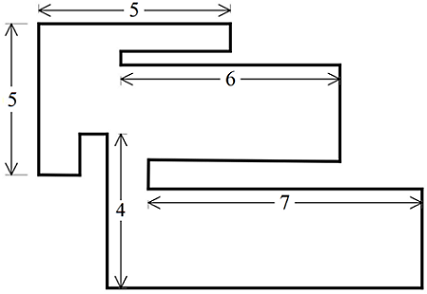# Do You Know The Ins And Outs Of This Figure?

Geometry Level 2The above shows a rectilinear figure (all its interior angles are either $90^\circ$ or $270^\circ$ only).

Calculate the perimeter of this figure.

×